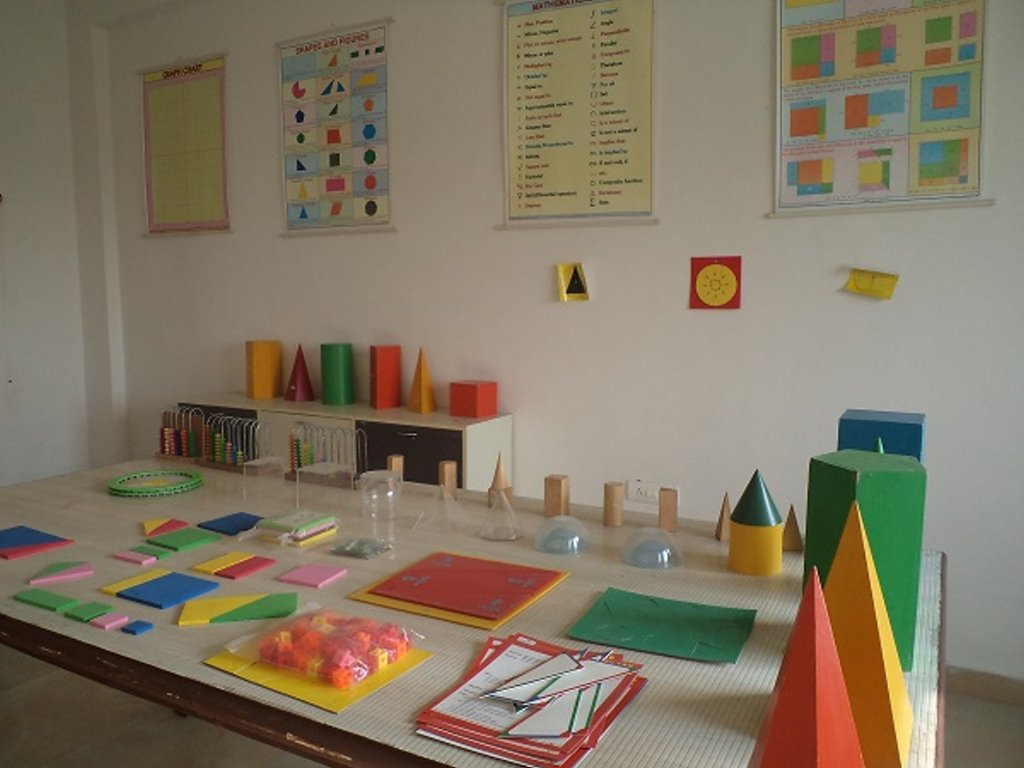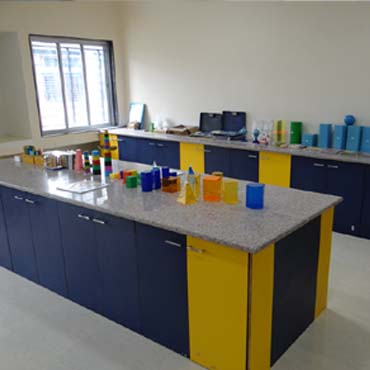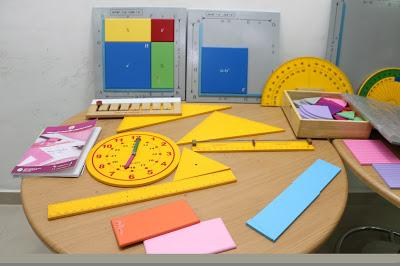• Affiliation No:-530034

• School Code:-40049

• UDISE Code: 06070106847

## Math's Lab

Mathematics Laboratory is a place where students can learn and explore mathematical concepts and verify mathematical facts and theorems through a variety of activities using different materials. These activities are carried out by the teacher and the student to explore, to learn, to stimulate interest and develop favorable attitude towards mathematics.The Maths Lab consists of more than 100 models in various branches of math’s like Algebra, Geometry, Arithmetic’s ,etc. which are of great help to students from K.G to 12th.The models are so designed to cater to the needs of all segments of teachers and learners in verifying formulae results and applications etc., irrespective of curriculum. A math lab is a classroom or designated space to teach math lessons in an interactive and engaging way. Appropriate equipment enable students to learn and improve their mathematical abilities. In a school, education center setting up a math lab in preparation for lessons is essential so that the students and professor have the tools necessary to work through the course material. The Mathematics Laboratory is a place, rich in manipulative material, to which children have ready access to handle them, perform mathematical experiments, play mathematical games, solve mathematical puzzles and become involved in other activities.